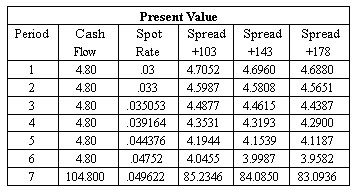Fixed Income I

Reading 44. Introduction to Fixed-Income Valuation

Learning Outcome Statements

k. compare, calculate, and interpret yield spread measures.

CFA Curriculum, 2020, Volume 5

### Why should I choose AnalystNotes?

AnalystNotes specializes in helping candidates pass. Period.

A bond's yield-to-maturity can be separated into a benchmark and a spread.

• Benchmark rates are usually yields-to-maturity on government bonds or fixed rates on interest rate swaps.

Changes in benchmark rates (risk-free rate of return) capture macroeconomic factors that affect all bonds in the market: inflation, economic growth, foreign exchange rates, and monetary and fiscal policy.

• Changes in spreads (risk premium component) typically capture microeconomic factors that affect a particular bond: credit risk, liquidity and tax effects.

• G spread: the spread over or under a government bond rate, also known as the nominal spread. For example, suppose a 10-year, 8%-coupon bond is selling at \$104.19, yielding 7.40%. The 10-year Treasury bond (6% coupon rate) has a YTM of 6.00%. Therefore, the G spread is 7.40% - 6.00% = 1.40%, or 140 basis points.

• I spread: the yield spread over or under the standard swap rate in that currency of the same tenor.

• Z spread (zero volatility spread): the constant yield spread over the benchmark spot curve such that the present value of the cash flows matches the price of the bond.

• OAS (option-adjusted spread): Z spread - option value. It is used for bonds with embedded options.

• For callable bonds and bonds with prepayment options (e.g., most mortgage-backed and asset-backed securities), option cost > 0 and thus OAS < Z-Spread. The option cost is positive since the options are a detrimental to bondholders.
• For putable bonds, option cost < 0 and thus OAS > Z-Spread.

Example

Phil Deter was interested in purchasing a non-Treasury bond for 110.2950. Given the Treasury spot rate data below, and assuming that the non-Treasury bond had a coupon of 9.60%, what is the likely Z-spread that Phil will earn over the duration of his investment?It is important to add all of the cash flows for each bond (discounted at the appropriate spot rate) and compare these to the purchase price by trial-and-error.

The bond with a spread of 143 Basis Points has a purchase price of 4.70 + 4.58 + 4.46 + 4.32 + 4.15 + 4.00 + 84.08 = 110.2950. Since this purchase price corresponds with the bond corresponding with Phil's interest, the appropriate spread must be 143 Basis Points.

###### shasha

Two critical assumptions of the valuation model for OAS: 1) interest rate volatility, the higher, the lower OAS; 2) yield curve on which the benchmark is based on.

###### shasha

option cost is the difference between the spread earned in a constant interest rate environment and the spread earned with a volatility assumption on interest rates. so could we say high option cost means a big "cost" when interest volatility happened according to the valuation model's assumption?

###### shasha

well, may it be saying: Spread IS +xxx bp, not Spread PLUS xxx bp? anyway, not a big deal.

###### shasha

the head line of above table should be read: "spot rate + bp" instead of "spread + bp". and obviously it's semiannual bond.

###### reganbaha

The line on the table is correct. It is saying that the spread 'is' +143 bps, not spread +143 bps.

###### johntan1979

For the Phil Deter example, is adding up all the numbers to find the answer the only way? Drives me nuts! :(